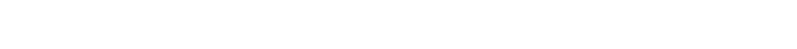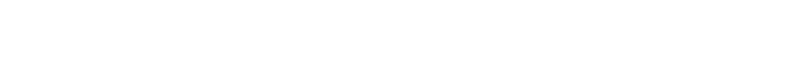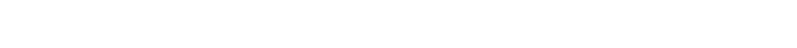## Search

Machine learning Filter

# What is LDA: Linear Discriminant Analysis for Machine Learning

10262Linear Discriminant Analysis or LDA is a dimensionality reduction technique. It is used as a pre-processing step in Machine Learning and applications of pattern classification. The goal of LDA is to project the features in higher dimensional space onto a lower-dimensional space in order to avoid the curse of dimensionality and also reduce resources and dimensional costs.

The original technique was developed in the year 1936 by Ronald A. Fisher and was named Linear Discriminant or Fisher's Discriminant Analysis. The original Linear Discriminant was described as a two-class technique. The multi-class version was later generalized by C.R Rao as Multiple Discriminant Analysis. They are all simply referred to as the Linear Discriminant Analysis.

LDA is a supervised classification technique that is considered a part of crafting competitive machine learning models. This category of dimensionality reduction is used in areas like image recognition and predictive analysis in marketing.

### What is Dimensionality Reduction?

The techniques of dimensionality reduction are important in applications of Machine Learning, Data Mining, Bioinformatics, and Information Retrieval. The main agenda is to remove the redundant and dependent features by changing the dataset onto a lower-dimensional space.

In simple terms, they reduce the dimensions (i.e. variables) in a particular dataset while retaining most of the data.

Multi-dimensional data comprises multiple features having a correlation with one another. You can plot multi-dimensional data in just 2 or 3 dimensions with dimensionality reduction. It allows the data to be presented in an explicit manner which can be easily understood by a layman.

### What are the limitations of Logistic Regression?

Logistic Regression is a simple and powerful linear classification algorithm. However, it has some disadvantages which have led to alternate classification algorithms like LDA. Some of the limitations of Logistic Regression are as follows:

• Two-class problems – Logistic Regression is traditionally used for two-class and binary classification problems. Though it can be extrapolated and used in multi-class classification, this is rarely performed. On the other hand, Linear Discriminant Analysis is considered a better choice whenever multi-class classification is required and in the case of binary classifications, both logistic regression and LDA are applied.
• Unstable with Well-Separated classes – Logistic Regression can lack stability when the classes are well-separated. This is where LDA comes in.
• Unstable with few examples – If there are few examples from which the parameters are to be estimated, logistic regression becomes unstable. However, Linear Discriminant Analysis is a better option because it tends to be stable even in such cases.

### How to have a practical approach to an LDA model?

Consider a situation where you have plotted the relationship between two variables where each color represents a different class. One is shown with a red color and the other with blue.If you are willing to reduce the number of dimensions to 1, you can just project everything to the x-axis as shown below:This approach neglects any helpful information provided by the second feature. However, you can use LDA to plot it. The advantage of LDA is that it uses information from both the features to create a new axis which in turn minimizes the variance and maximizes the class distance of the two variables.### How does LDA work?

LDA focuses primarily on projecting the features in higher dimension space to lower dimensions. You can achieve this in three steps:

• Firstly, you need to calculate the separability between classes which is the distance between the mean of different classes. This is called the between-class variance.• Secondly, calculate the distance between the mean and sample of each class. It is also called the within-class variance.• Finally, construct the lower-dimensional space which maximizes the between-class variance and minimizes the within-class variance. P is considered as the lower-dimensional space projection, also called Fisher’s criterion.### How are LDA models represented?

The representation of LDA is pretty straight-forward. The model consists of the statistical properties of your data that has been calculated for each class. The same properties are calculated over the multivariate Gaussian in the case of multiple variables. The multivariates are means and covariate matrix.

Predictions are made by providing the statistical properties into the LDA equation. The properties are estimated from your data. Finally, the model values are saved to file to create the LDA model.

### How do LDA models learn?

• Each variable in the data is shaped in the form of a bell curve when plotted,i.e. Gaussian.
• The values of each variable vary around the mean by the same amount on the average,i.e. each attribute has the same variance.

The LDA model is able to estimate the mean and variance from your data for each class with the help of these assumptions.

The mean value of each input for each of the classes can be calculated by dividing the sum of values by the total number of values:

Mean =Sum(x)/Nk

where Mean = mean value of x for class
N = number of
k = number of
Sum(x) = sum of values of each input x.

The variance is computed across all the classes as the average of the square of the difference of each value from the mean:

Σ²=Sum((x - M)²)/(N - k)

where  Σ² = Variance across all inputs x.
N = number of instances.
k = number of classes.
Sum((x - M)²) = Sum of values of all (x - M)².
M = mean for input x.

### How does an LDA model make predictions?

LDA models use Bayes’ Theorem to estimate probabilities. They make predictions based upon the probability that a new input dataset belongs to each class. The class which has the highest probability is considered the output class and then the LDA makes a prediction.

The prediction is made simply by the use of Bayes’ Theorem which estimates the probability of the output class given the input. They also make use of the probability of each class and the probability of the data belonging to each class:

P(Y=x|X=x)  = [(Plk * fk(x))] / [(sum(PlI * fl(x))]

Where x = input.
k = output class.
Plk = Nk/n or base probability of each class observed in the training data. It is also called prior probability in Bayes’ Theorem.
fk(x) = estimated probability of x belonging to class k.

The f(x) is plotted using a Gaussian Distribution function and then it is plugged into the equation above and the result we get is the equation as follows:

Dk(x) = x∗(mean/Σ²) – (mean²/(2*Σ²)) + ln(PIk)

The Dk(x) is called the discriminant function for class k given input x, mean,  Σ² and Plk are all estimated from the data and the class is calculated as having the largest value, will be considered in the output classification.

### How to prepare data from LDA?

Some suggestions you should keep in mind while preparing your data to build your LDA model:

• LDA is mainly used in classification problems where you have a categorical output variable. It allows both binary classification and multi-class classification.
• The standard LDA model makes use of the Gaussian Distribution of the input variables. You should check the univariate distributions of each attribute and transform them into a more Gaussian-looking distribution. For example, for the exponential distribution, use log and root function and for skewed distributions use BoxCox.
• Outliers can skew the primitive statistics used to separate classes in LDA, so it is preferable to remove them.
• Since LDA assumes that each input variable has the same variance, it is always better to standardize your data before using an LDA model. Keep the mean to be 0 and the standard deviation to be 1.

### How to implement an LDA model from scratch?

You can implement a Linear Discriminant Analysis model from scratch using Python. Let’s start by importing the libraries that are required for the model:

```from sklearn.datasets import load_wine
import pandas as pd
import numpy as np
np.set_printoptions(precision=4)
from matplotlib import pyplot as plt
import seaborn as sns
sns.set()
from sklearn.preprocessing import LabelEncoder
from sklearn.tree import DecisionTreeClassifier
from sklearn.model_selection import train_test_split
from sklearn.metrics import confusion_matrix```

Since we will work with the wine dataset, you can obtain it from the UCI machine learning repository. The scikit-learn library in Python provides a wrapper function for downloading it:

```wine_info = load_wine()
X = pd.DataFrame(wine_info.data, columns=wine_info.feature_names)
y = pd.Categorical.from_codes(wine_info.target, wine_info.target_names)```

The wine dataset comprises of 178 rows of 13 columns each:

`X.shape`
`(178, 13)`

The attributes of the wine dataset comprise of various characteristics such as alcohol content of the wine, magnesium content, color intensity, hue and many more:

`X.head()`The wine dataset contains three different kinds of wine:

```wine_info.target_names
array(['class_0', 'class_1', 'class_2'], dtype='<U7')```

Now we create a DataFrame which will contain both the features and the content of the dataset:

`df = X.join(pd.Series(y, name='class'))`

We can divide the process of Linear Discriminant Analysis into 5 steps as follows:

Step 1 - Computing the within-class and between-class scatter matrices.
Step 2 - Computing the eigenvectors and their corresponding eigenvalues for the scatter matrices.
Step 3 - Sorting the eigenvalues and selecting the top k.
Step 4 - Creating a new matrix that will contain the eigenvectors mapped to the k eigenvalues.
Step 5 - Obtaining new features by taking the dot product of the data and the matrix from Step 4.

### Within-class scatter matrix

To calculate the within-class scatter matrix, you can use the following mathematical expression:where, c = total number of distinct classes andwhere, x = a sample (i.e. a row).
n = total number of samples within a given class.

Now we create a vector with the mean values of each feature:

```feature_means1 = pd.DataFrame(columns=wine_info.target_names)
for c, rows in df.groupby('class'):
feature_means1[c] = rows.mean()
feature_means1```The mean vectors (mi ) are now plugged into the above equations to obtain the within-class scatter matrix:

```withinclass_scatter_matrix = np.zeros((13,13))
for c, rows in df.groupby('class'):
rows = rows.drop(['class'], axis=1)

s = np.zeros((13,13))
for index, row in rows.iterrows():
x, mc = row.values.reshape(13,1),
feature_means1[c].values.reshape(13,1)

s += (x - mc).dot((x - mc).T)

withinclass_scatter_matrix += s```

### Between-class scatter matrix

We can calculate the between-class scatter matrix using the following mathematical expression:where,and```feature_means2 = df.mean()
betweenclass_scatter_matrix = np.zeros((13,13))
for c in feature_means1:
n = len(df.loc[df['class'] == c].index)
mc, m = feature_means1[c].values.reshape(13,1),
feature_means2.values.reshape(13,1)
betweenclass_scatter_matrix += n * (mc - m).dot((mc - m).T)```

Now we will solve the generalized eigenvalue problem to obtain the linear discriminants for:```eigen_values, eigen_vectors =
np.linalg.eig(np.linalg.inv(withinclass_scatter_matrix).dot(betweenclass_scatter_matrix))```

We will sort the eigenvalues from the highest to the lowest since the eigenvalues with the highest values carry the most information about the distribution of data is done. Next, we will first k eigenvectors. Finally, we will place the eigenvalues in a temporary array to make sure the eigenvalues map to the same eigenvectors after the sorting is done:

```eigen_pairs = [(np.abs(eigen_values[i]), eigen_vectors[:,i]) for i in range(len(eigen_values))]
eigen_pairs = sorted(eigen_pairs, key=lambda x: x, reverse=True)
for pair in eigen_pairs:
print(pair)```
```237.46123198302251
46.98285938758684
1.4317197551638386e-14
1.2141209883217706e-14
1.2141209883217706e-14
8.279823065850476e-15
7.105427357601002e-15
6.0293733655173466e-15
6.0293733655173466e-15
4.737608877108813e-15
4.737608877108813e-15
2.4737196789039026e-15
9.84629525010022e-16```

Now we will transform the values into percentage since it is difficult to understand how much of the variance is explained by each component.

```sum_of_eigen_values = sum(eigen_values)
print('Explained Variance')
for i, pair in enumerate(eigen_pairs):
print('Eigenvector {}: {}'.format(i, (pair/sum_of_eigen_values).real))```
```Explained Variance
Eigenvector 0: 0.8348256799387275
Eigenvector 1: 0.1651743200612724
Eigenvector 2: 5.033396012077518e-17
Eigenvector 3: 4.268399397827047e-17
Eigenvector 4: 4.268399397827047e-17
Eigenvector 5: 2.9108789097898625e-17
Eigenvector 6: 2.498004906118145e-17
Eigenvector 7: 2.119704204950956e-17
Eigenvector 8: 2.119704204950956e-17
Eigenvector 9: 1.665567688286435e-17
Eigenvector 10: 1.665567688286435e-17
Eigenvector 11: 8.696681541121664e-18
Eigenvector 12: 3.4615924706522496e-18```

First, we will create a new matrix W using the first two eigenvectors:

W_matrix = np.hstack((eigen_pairs.reshape(13,1), eigen_pairs.reshape(13,1))).real

Next, we will save the dot product of X and W into a new matrix Y:

Y = X∗W

where, X = n x d matrix with n sample and d dimensions.
Y = n x k matrix with n sample and k dimensions.

In simple terms, Y is the new matrix or the new feature space.

`X_lda = np.array(X.dot(W_matrix))`

Our next work is to encode every class a member in order to incorporate the class labels into our plot. This is done because matplotlib cannot handle categorical variables directly.

Finally, we plot the data as a function of the two LDA components using different color for each class:

```plt.xlabel('LDA1')
plt.ylabel('LDA2')
plt.scatter(
X_lda[:,0],
X_lda[:,1],
c=y,
cmap='rainbow',
alpha=0.7,
edgecolors='b'
)```
`<matplotlib.collections.PathCollection at 0x7fd08a20e908>`### How to implement LDA using scikit-learn?

For implementing LDA using scikit-learn, let’s work with the same wine dataset. You can also obtain it from the  UCI machine learning repository.

You can use the predefined class LinearDiscriminant Analysis made available to us by the scikit-learn library to implement LDA rather than implementing from scratch every time:

```from sklearn.discriminant_analysis import LinearDiscriminantAnalysis
lda_model = LinearDiscriminantAnalysis()
X_lda = lda_model.fit_transform(X, y)```

To obtain the variance corresponding to each component, you can access the following property:

`lda.explained_variance_ratio_`
`array([0.6875, 0.3125])`

Again, we will plot the two LDA components just like we did before:

```plt.xlabel('LDA1')
plt.ylabel('LDA2')
plt.scatter(
X_lda[:,0],
X_lda[:,1],
c=y,
cmap='rainbow',
alpha=0.7,
edgecolors='b'
)```
`<matplotlib.collections.PathCollection at 0x7fd089f60358>`### Linear Discriminant Analysis vs PCA

Below are the differences between LDA and PCA:

• PCA ignores class labels and focuses on finding the principal components that maximizes the variance in a given data. Thus it is an unsupervised algorithm. On the other hand, LDA is a supervised algorithm that intends to find the linear discriminants that represents those axes which maximize separation between different classes.
• LDA performs better multi-class classification tasks than PCA. However, PCA performs better when the sample size is comparatively small. An example would be comparisons between classification accuracies that are used in image classification.
• Both LDA and PCA are used in case of dimensionality reduction. PCA is first followed by LDA.Let us create and fit an instance of the PCA class:

```from sklearn.decomposition import PCA
pca_class = PCA(n_components=2)
X_pca = pca.fit_transform(X, y)```

Again, to view the values in percentage for a better understanding, we will access the explained_variance_ratio_ property:

`pca.explained_variance_ratio_`
`array([0.9981, 0.0017])`

Clearly, PCA selected the components which will be able to retain the most information and ignores the ones which maximize the separation between classes.

```plt.xlabel('PCA1')
plt.ylabel('PCA2')
plt.scatter(
X_pca[:,0],
X_pca[:,1],
c=y,
cmap='rainbow',
alpha=0.7,
edgecolors='b```Now to create a classification model using the LDA components as features, we will divide the data into training datasets and testing datasets:

`X_train, X_test, y_train, y_test = train_test_split(X_lda, y, random_state=1)`

The next thing we will do is create a Decision Tree. Then, we will predict the category of each sample test and create a confusion matrix to evaluate the LDA model’s performance:

```data = DecisionTreeClassifier()
data.fit(X_train, y_train)
y_pred = data.predict(X_test)
confusion_matrix(y_test, y_pred)```
```array([[18,  0,  0],
[ 0, 17,  0],
[ 0,  0, 10]])```

So it is clear that the Decision Tree Classifier has correctly classified everything in the test dataset.

### What are the extensions to LDA?

LDA is considered to be a very simple and effective method, especially for classification techniques. Since it is simple and well understood, so it has a lot of extensions and variations:

• Quadratic Discriminant Analysis(QDA) – When there are multiple input variables, each of the class uses its own estimate of variance and covariance.
• Flexible Discriminant Analysis(FDA) – This technique is performed when a non-linear combination of inputs is used as splines.
• Regularized Discriminant Analysis(RDA) – It moderates the influence of various variables in LDA by regularizing the estimate of the covariance.

### Real-Life Applications of LDA

Some of the practical applications of LDA are listed below:

• Face Recognition – LDA is used in face recognition to reduce the number of attributes to a more manageable number before the actual classification. The dimensions that are generated are a linear combination of pixels that forms a template. These are called Fisher’s faces.
• Medical – You can use LDA to classify the patient disease as mild, moderate or severe. The classification is done upon the various parameters of the patient and his medical trajectory.
• Customer Identification – You can obtain the features of customers by performing a simple question and answer survey. LDA helps in identifying and selecting which describes the properties of a group of customers who are most likely to buy a particular item in a shopping mall.

Summary

Let us take a look at the topics we have covered in this article:

• Dimensionality Reduction and need for LDA
• Working of an LDA model
• Representation, Learning, Prediction and preparing data in LDA
• Implementation of an LDA model
• Implementation of LDA using scikit-learn
• LDA vs PCA
• Extensions and Applications of LDA

The Linear Discriminant Analysis in Python is a very simple and well-understood approach of classification in machine learning. Though there are other dimensionality reduction techniques like Logistic Regression or PCA, but LDA is preferred in many special classification cases. If you want to be an expert in machine learning, knowledge of Linear Discriminant Analysis would lead you to that position effortlessly. Enrol in our  Data Science and Machine Learning Courses for more lucrative career options in this landscape and become a certified Data Scientist.### Priyankur Sarkar

Data Science Enthusiast

Priyankur Sarkar loves to play with data and get insightful results out of it, then turn those data insights and results in business growth. He is an electronics engineer with a versatile experience as an individual contributor and leading teams, and has actively worked towards building Machine Learning capabilities for organizations.

## Types of Probability Distributions Every Data Science Expert Should know

9709
Types of Probability Distributions Every Data Scie...

Data Science has become one of the most popular in... Read More